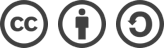## Scala基础知识2

2021-02-26

Ideal中如何关联scala源代码

ideal中用Attach sources

``````scaladoc -d  ./doc hello.scala
``````

java中的常量定义 final int a =10 ;

Scala变量定义 ：var a:Int=10;

Constant 打勾

``````def main(args: Array[String]): Unit = {
println(str)
}

hcb
``````

java基本类型8种：char,byte,short,int,long,float,double,boolean

Scala 数据类型：都是Any的子类， 分为数值类型AnyVal，引用类型AnyRef

Unit类型：无值：与void等同， 只有一个实例值（）

Null类型：一个实例值null

Nothing类型：是任何类型的子类型，异常发生时返回。

for循环

``````for (i<- 1 to 3){
println(i)
}

for (i<- 1 to 3;j <- 10 to 13){
println(i +"|||"+j)
}

#倒序
for (i<- 1 to 3 reverse){
println(i)
}
3
2
1

#contine 终止本次循环，继续下一次循环
#scala中 没有这两个关键字，要采用抛出异常的方式来处理。
#Breaks.break
``````

``````def hi(s:String*):Unit={
println(s)
}

hi()
hi("11")

//输出结果
List()
WrappedArray(11)
``````

``````   def hi(s:String*):Unit={
println(s)
}

val ff: Seq[String] => Unit = hi
print(ff)

``````

`````` def sum(a:Int,b:Int,op:(Int,Int)=>Int):Int={
op(a,b)
}
def op(a:Int,b:Int):Int={
a+b
}
print(sum(1,2,op))

``````

``````def sum():()=>Unit={
def sum1():Unit={
println("sum1 invoke")
}
sum1 _
}
//调用 ，嵌套函数
sum()()

//output result
sum1 invoke
``````

javac -d . Hello.java

import 导类不是导包。

import java.util._ //导入包下的所有类

``````import  java.sql.{Data=>,Array=>,_}
``````

IOC控制反转